# Class 12 Maths NCERT Solutions for Chapter 6 Application of Derivatives Exercise 6.1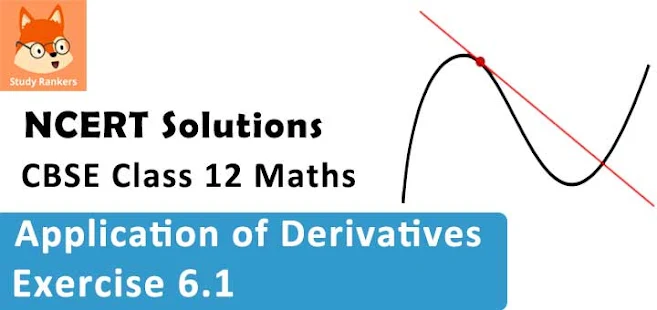### Determinants Miscellaneous Exercise Solutions

1. Find the rate of change of the area of a circle with respect to its radius r when
(i) r = 3 cm
(ii) r = 4 cm

Solution

The area of a circle (A) with radius (r) is given by,
A = πr2
Now, the rate of change of the area with respect to its radius is given by, dA/dr = d/dr(πr2) = 2πr
(i) When r = 3 cm,
dA/dr = 2π(3) = 6π
Hence, the area of the circle is changing at the rate of 6π cm2/cm when its radius is 3 cm.

(ii) When r = 4 cm
dA/dr = 2π(3) = 8π
Hence, the area of the circle is changing at the rate of 8π cm2/cm when its radius is 4 cm.

2. The volume of a cube is increasing at the rate of 8 cm3/s. How fast is the surface area increasing when the length of an edge is 12 cm?

Solution

Let x be the length of a side, V be the volume , and s be the surface area of the cube.
Then, V = x3 and S = 6x2 where x is a function of time t.
It is given that dv/dt = 8cm cm3/s  .
Then, by using the chain rule, we have :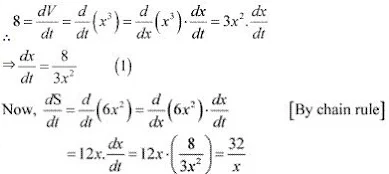Thus, when x = 12 cm, ds/dt = 32/12  cm2/s = 8/3 cm2/s.
Hence, if the length of the edge of the cube is 12 cm, then the surface area is increasing at the rat of 8/3 cm2/s

3. The radius of a circle is increasing uniformly at the rate of 3 cm/s. Find the rate at which the area of the circle is increasing when the radius is 10 cm.

Solution

The area of a circle (A) with radius (r) is given by,
A = πr2
Now, the rate of change of area (A) with respect to time (t) is given by,
dA/dt = d/dr(πr2) .dr/dt = 2πr dr/dt  [By chain rule]
It is given that,
dr/dt = 3 cm/s
∴ dA/dt = 2πr(3) = 6πr
Thus, when r = 10 cm,
dA/dt = 6π(10) = 60π cm2 /s
Hence, the rate at which the area of the circle is increasing when the radius is 10 cm is 60π cm2/s.

4. An edge of a variable cube is increasing at the rate of 3 cm/s. How fast is the volume of the cube increasing when the edge is 10 cm long?

Solution

Let x be the length of a side and V be the volume of the cube. Then,
V = x3 .
∴ dV/dt = 3x2 . dx/dt  (By chain rule)
It is given that,
dx/dt = 3 cm/s
∴ dV/dt = 3x2 (3) = 9x2
Thus, when x = 10 cm,
dV/dt =  9(10)2 = 900 cm3/s
Hence, the volume of the cube is increasing at the rate of 900 cm3/s when the edge is 10 cm long.

5. A stone is dropped into a quiet lake and waves move in circles at the speed of 5 cm/s. At the instant when the radius of the circular wave is 8 cm, how fast is the enclosed area increasing?

Solution

The area of a circle (A) with radius (r) is given by A = πr2 .
Therefore, the rate of change of area (A) with respect to time (t) is given by,
dA/dt = d/dt(πr2 ) = d/dr(πr2 ) dr/dt = 2πr dr/dt  [By chain rule]
It is given that dr/dt = 5 cm/s .
Thus, when r = 8 cm,
dA/dt = 2π (8)(5) = 80π
Hence, when the radius of the circular wave is 8 cm, the enclosed area is increasing at the rate of 80π cm2/s.

6.  The radius of a circle is increasing at the rate of 0.7 cm/s. What is the rate of increase of its circumference?

Solution

The circumference of a circle (C) with radius (r) is given by
C = 2πr .
Therefore, the rate of change of circumference (C) with respect to time (t) is given by,
dC/dt = dC/dr . dr/dt (By chain rule)
= d/dr (2πr ) dr/dt
= 2π . dr/dt
It is given that dr/dt = 0.7 cm/s.
Hence, the rate of increase of the circumference is 2π(0.7) = 1.4π cm/s.

7. The length x of a rectangle is decreasing at the rate of 5 cm/minute and the width y is increasing at the rate of 4 cm/minute. When x = 8 cm and y = 6 cm, find the rates of change of (a) the perimeter, and (b) the area of the rectangle.

Solution

Since the length (x) is decreasing at the rate of 5 cm/minute and the width(y) is increasing at the rate of 4 cm/minute, we have :
dx/dt = -5 cm/min and dy/dt = 4 cm/min
(a) The perimeter (p) of a rectangle is given by,
P = 2(x + y)
∴ dP/dt = 2(dx/dt + dy/dt) = 2(-5 + 4) = -2 cm/min
Hence, the perimeter is decreasing at the rate of 2 cm/min.
(b) The area (A) of a rectangle is given by,
A = x × y
∴ dA/dt = dx/dt . y + x. dy/dt = -5y + 4x
When x = 8 cm and y= 6 cm, dA/dt = (-5 × 6 + 4 × 8) cm2/min = 2 cm2/min
Hence, the area of the rectangle is increasing at the rate of 2 cm2/min.

8. A balloon, which always remains spherical on inflation, is being inflated by pumping in 900 cubic centimetres of gas per second. Find the rate at which the radius of the balloon increases when the radius is 15 cm.

Solution

The volume of a sphere (V) with radius (r) is given by,
V = 4/3(πr3 )
∴ Rate of change of volume (V) with respect to time (t) is given by,
dV/dt = dV/dr . dr/dt  [By chain rule]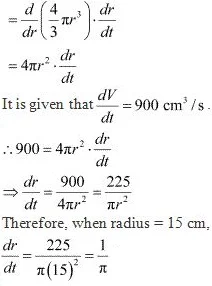Hence, the rate at which the radius of the balloon increases when the radius is 15 cm is 1/π cm/s.

9. A balloon, which always remains spherical has a variable radius. Find the rate at which its volume is increasing with the radius when the later is 10 cm.

Solution

The volume of a sphere (V) with radius (r) is given by V = (4/3)πr3 .
Rate of change of volume (V) with respect to its radius (r) is given by,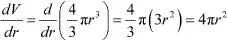Therefore, when radius = 10 cm,
dV/dr = 4π(10)2 = 400π
Hence, the volume of the balloon is increasing at the rate of 400π cm3/cm.

10. A ladder 5 m long is leaning against a wall. The bottom of the ladder is pulled along the ground, away from the wall, at the rate of 2 cm/s. How fast is its height on the wall decreasing when the foot of the ladder is 4 m away from the wall?

Solution

Let y m be the height of the wall at which the ladder touches. Also, let the foot of the ladder be x m away from the wall.
Then, by Pythagoras theorem, we have :
x2 + y2  = 25 [Length of the ladder = 5 m]
⇒ y = √(25 - x2 )
Then the rate of change of height(y) with respect to time (t) is given by,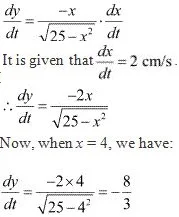Hence, the height of the ladder on the wall is decreasing at the rate of 8/3 cm/s.

11. A particle moves along the curve 6y = x3 +2. Find the points on the curve at which the y-coordinate is changing 8 times as fast as the x-coordinate.

Solution

The equation of the curve is given as :
6y = x3 +2
The rate of change of the position of the particle with respect to time (t) is given by,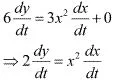When the y - coordinate of the particle changes 8 times as fast as the x - coordinate i.e., (dy/dt = 8 dx/dt), we have :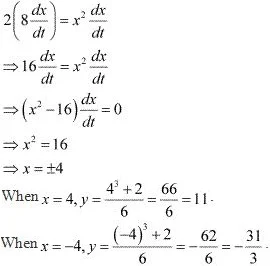Hence, the points required on the curve are (4, 11) and (-4, -31/3).

12. The radius of an air bubble is increasing at the rate 1/2 cm/s. At what rate is the volume of the bubble increasing when the radius is 1 cm?

Solution

The air bubble is in the shape of a sphere.
Now, the volume of an air bubble(V) with radius(r) is given by,
V = (4/3)πr3
The rate of change of volume (V) with respect to time (t) is given by,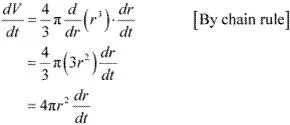It is given that dr/dt = 1/2 cm/s.
Therefore, when r = 1 cm,
dv/dt = 4π (1)2 (1/2) = 2π cm3/s
Hence, the rate at which the volume of the bubble increases is 2π cm3/s

13. A balloon, which always remains spherical, has a variable diameter 3/2 (2x+ 1) Find the rate of change of its volume with respect to x.

Solution

The volume of a sphere (V) with radius (r) is given by,
V = (4/3)πr3
It is given that :
Diameter = (3/2) (2x + 1)
⇒ r = (3/4) (2x + 1)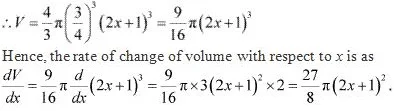14. Sand is pouring from a pipe at the rate of 12 cm3/s. The falling sand forms a cone on the ground in such a way that the height of the cone is always one-sixth of the radius of the base. How fast is the height of the sand cone increasing when the height is 4 cm?
Solution
The volume of a cone (V) with radius (r)  and height (h) is given by,
V = (1/3)πr3 h
It is given that,
h = (1/6)r
⇒ r = 6h
∴ V = (1/3)π(6h)2 h = 12πh3
The rate of change of volume with respect to time (t) is given by,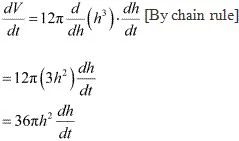It is also given that dV/dt = 12 cm3 /s.
Therefore, when h = 4 cm, we have :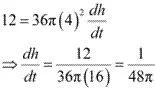Hence, when the height of the sand cone is 4 cm, its height is increasing at the rate of 1/48π  cm/s.

15. The total cost C(x) in Rupees associated with the production of x units of an item is given by C(x) = 0.007x3 – 0.003x2 + 15x + 4000.
Find the marginal cost when 17 units are produced
Solution
Marginal cost is the rate of change of total cost with respect to output.
∴ Marginal cost (MC) = dC/dx = 0.007 (3x2 ) - 0.003(2x) + 15
= 0.021x2 - 0.006x + 15
When x = 17, MC = 0.021(172) - 0.006 (17) + 15
= 0.021(289) - 0.006(17) + 15
= 6.069 - 0.102 + 15
= 20.967
Hence, when 17 units are produced, the marginal cost is Rs. 20.967

16. The total revenue in Rupees received from the sale of x units of a product is given by
R(x) = 13x2 + 26x + 15.
Find the marginal revenue when x = 7.
Solution
Marginal revenue is the rate of change of total revenue with respect to the number of units sold.
∴ Marginal Revenue (MR) = dR/dx = 13(2x) + 26 = 26x + 26
When x = 7,
MR = 26(7)  + 26 = 182 + 26 = 208
Hence, the required marginal revenue is Rs 208.

17. The rate of change of the area of a circle with respect to its radius r at r = 6 cm is
(A) 10π
(B) 12π
(C) 8π
(D) 11π
Solution
The area of a circle (A) with radius (r) is given by,
a = πr3
Therefore, the rate of change of the area with respect to its radius r is
dA/dr = d/dr (πr2 ) - 2πr
∴ When r = 6 cm,
dA/dr = 2π × 6 = 12π cm2/s
Hence, the required rate of change of the area of a circle is 12πcm2/s .